#### Conditional probability

A conditional probability is expressed as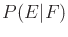for any two events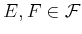and is called the probability of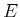, given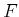.'' Its definition is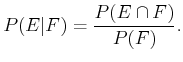(9.4)

Two events,and, are called independent if and only if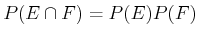; otherwise, they are called dependent. An important and sometimes misleading concept is conditional independence. Consider some third event,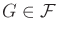. It might be the case thatandare dependent, but when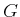is given, they become independent. Thus,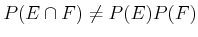; however,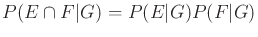. Such examples occur frequently in practice. For example,might indicate someone's height, andis their reading level. These will generally be dependent events because children are generally shorter and have a lower reading level. If we are given the person's age as an event, then height is no longer important. It seems intuitive that there should be no correlation between height and reading level once the age is given.

The definition of conditional probability, (9.4), imposes the constraint that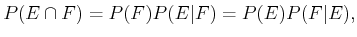(9.5)

which nicely relatesto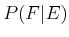. This results in Bayes' rule, which is a convenient way to swapand: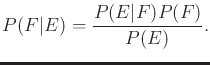(9.6)

The probability distribution,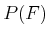, is referred to as the prior, andis the posterior. These terms indicate that the probabilities come before and afteris considered, respectively.

If all probabilities are conditioned on some event,, then conditional Bayes' rule arises, which only differs from (9.6) by placing the conditionon all probabilities: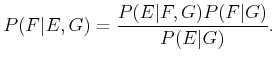(9.7)

Steven M LaValle 2020-08-14